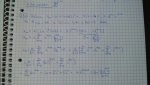# limit with sums

#### Vali

##### New member
Hi!

I have the following sequence $$\displaystyle (x_{n})_{n\geq 1}, \ x_{n}=ac+(a+ab)c^{2}+...+(a+ab+...+ab^{n})c^{n+1}$$
Also I know that $$\displaystyle a,b,c\in \mathbb{R}$$ and $$\displaystyle |c|<1,\ b\neq 1, \ |bc|<1$$
I need to find the limit of $$\displaystyle x_{n}$$.

My attempt is in the picture.The result should be $$\displaystyle \frac{ac}{(1-bc)(1-c)}$$
I miss something at these two sums which are geometric progressions.Each sum should start with $$\displaystyle 1$$ but why ? If k starts from 0 results the first terms are $$\displaystyle bc$$ and $$\displaystyle c$$ right?#### Vali

##### New member
I'm stupid, I got the correct answer.I just needed to solve some little calculations.I don't know I thought I'm wrong..
Thanks!